# 1 Signal Encoding Techniques Data and Computer Communications

• Slides: 38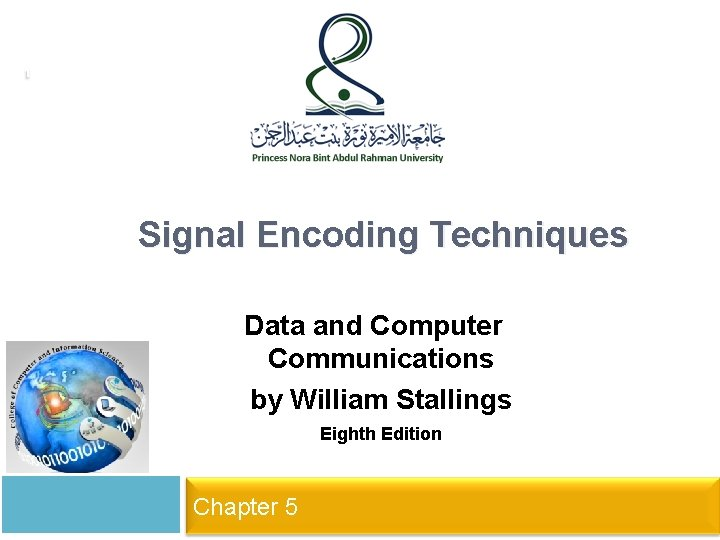1 Signal Encoding Techniques Data and Computer Communications by William Stallings Eighth Edition Networks and Communication Department Click to 5 edit Master subtitle style Chapter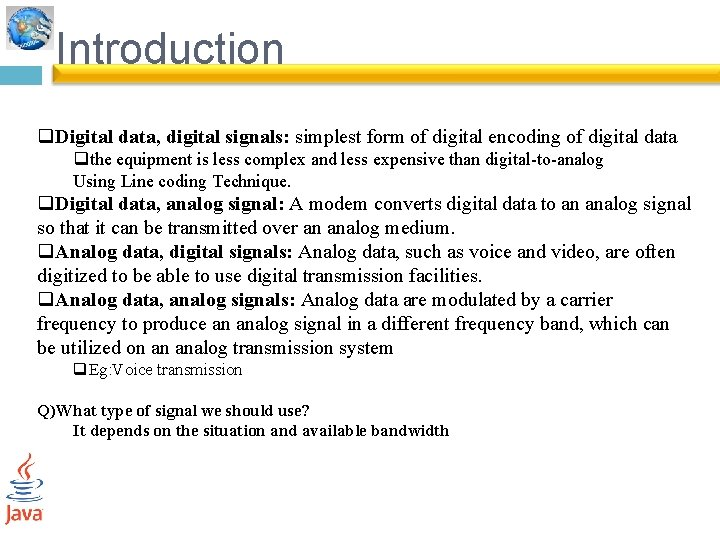Introduction q. Digital data, digital signals: simplest form of digital encoding of digital data qthe equipment is less complex and less expensive than digital-to-analog Using Line coding Technique. q. Digital data, analog signal: A modem converts digital data to an analog signal so that it can be transmitted over an analog medium. q. Analog data, digital signals: Analog data, such as voice and video, are often digitized to be able to use digital transmission facilities. q. Analog data, analog signals: Analog data are modulated by a carrier frequency to produce an analog signal in a different frequency band, which can be utilized on an analog transmission system q. Eg: Voice transmission Q)What type of signal we should use? It depends on the situation and available bandwidth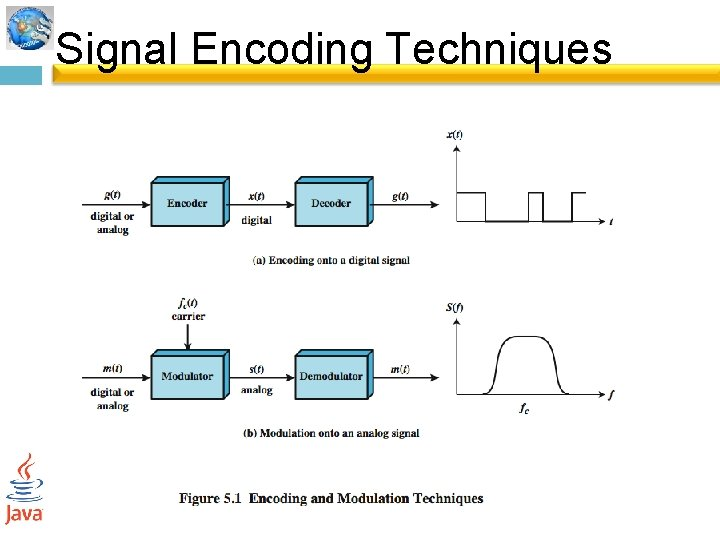Signal Encoding Techniques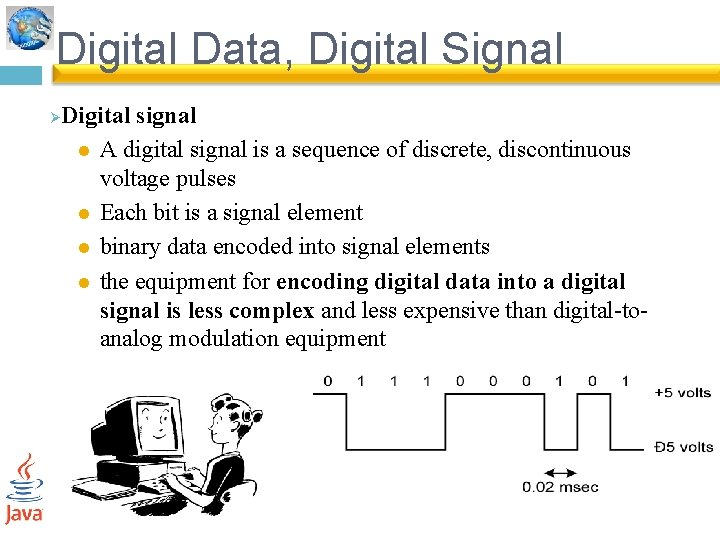Digital Data, Digital Signal Ø Digital signal l A digital signal is a sequence of discrete, discontinuous voltage pulses l Each bit is a signal element l binary data encoded into signal elements l the equipment for encoding digital data into a digital signal is less complex and less expensive than digital-toanalog modulation equipment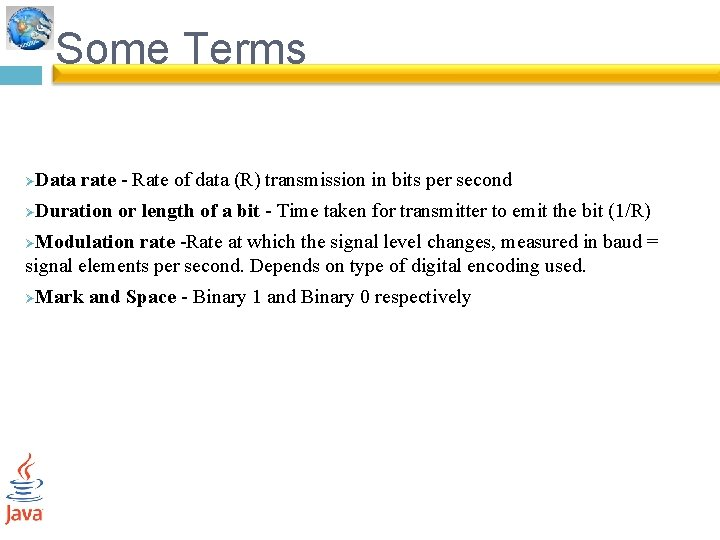Some Terms Data rate - Rate of data (R) transmission in bits per second Ø Duration or length of a bit - Time taken for transmitter to emit the bit (1/R) Ø Modulation rate -Rate at which the signal level changes, measured in baud = signal elements per second. Depends on type of digital encoding used. Ø Mark and Space - Binary 1 and Binary 0 respectively ØSome Terms Line coding schemes: Unipolar – It uses only one voltage level Ø Polar – use two voltage levels , one positive and the other one negative Ø Bipolar-use three voltage levels , positive voltage , 0 voltage and negative voltage Ø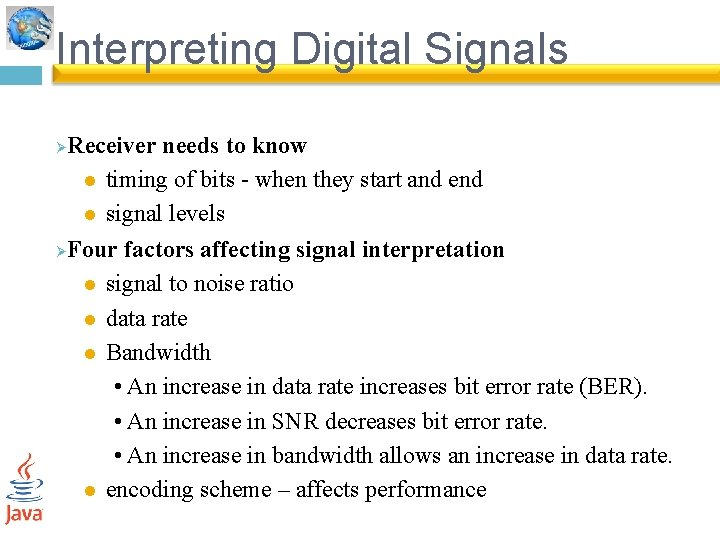Interpreting Digital Signals Receiver needs to know l timing of bits - when they start and end l signal levels ØFour factors affecting signal interpretation l signal to noise ratio l data rate l Bandwidth • An increase in data rate increases bit error rate (BER). • An increase in SNR decreases bit error rate. • An increase in bandwidth allows an increase in data rate. l encoding scheme – affects performance Ø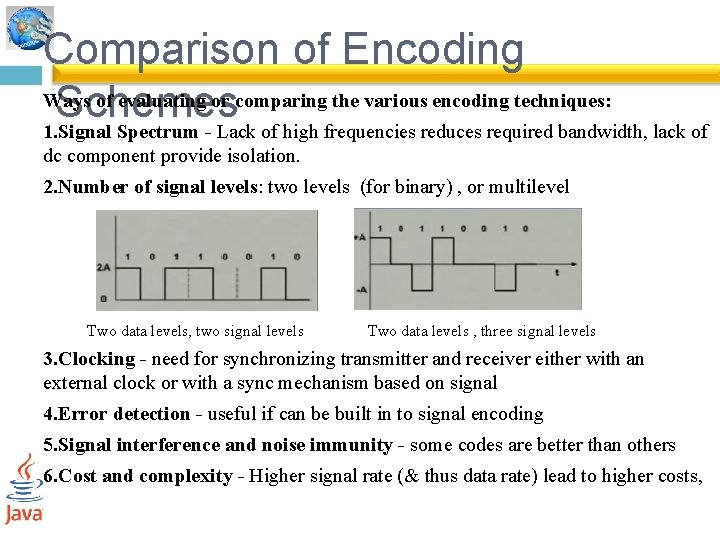Comparison of Encoding Ways of evaluating or comparing the various encoding techniques: Schemes 1. Signal Spectrum - Lack of high frequencies reduces required bandwidth, lack of dc component provide isolation. 2. Number of signal levels: two levels (for binary) , or multilevel Two data levels, two signal levels Two data levels , three signal levels 3. Clocking - need for synchronizing transmitter and receiver either with an external clock or with a sync mechanism based on signal 4. Error detection - useful if can be built in to signal encoding 5. Signal interference and noise immunity - some codes are better than others 6. Cost and complexity - Higher signal rate (& thus data rate) lead to higher costs,Encoding Schemes Encoding scheme is the mapping from data bits to signal element. They include: 1. Nonreturn to Zero-Level (NRZ-L) 2. Nonreturn to Zero Inverted (NRZI) 3. Bipolar -AMI 4. Pseudoternary 5. Manchester 6. Differential Manchester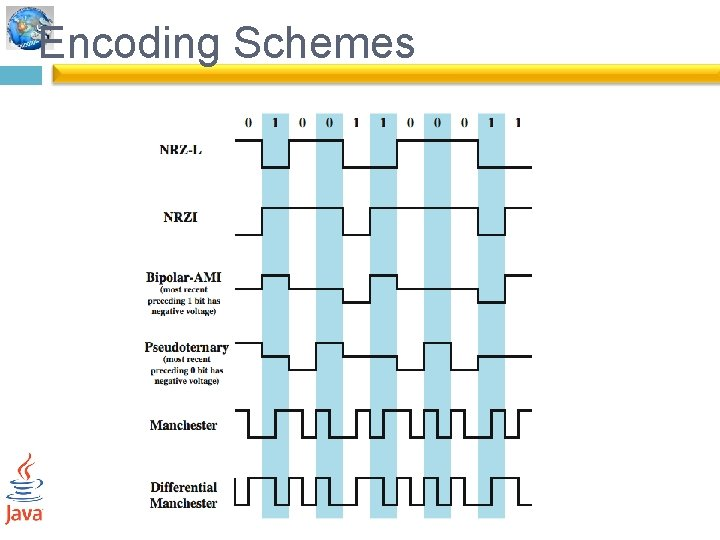Encoding Schemes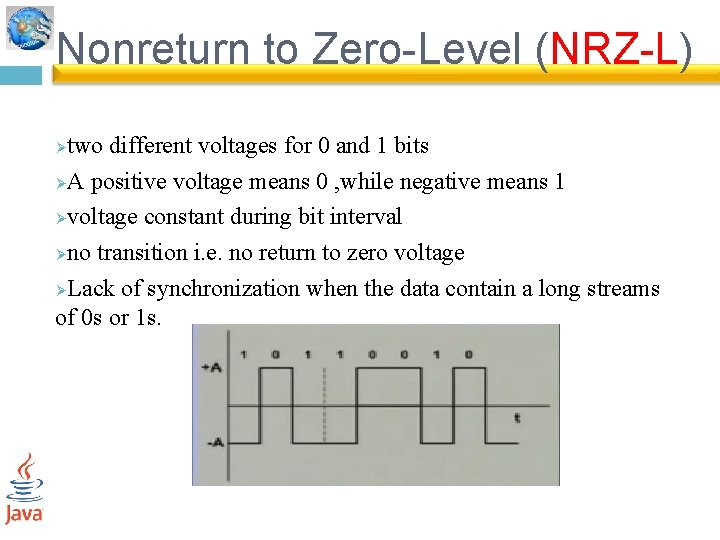Nonreturn to Zero-Level (NRZ-L) two different voltages for 0 and 1 bits ØA positive voltage means 0 , while negative means 1 Øvoltage constant during bit interval Øno transition i. e. no return to zero voltage ØLack of synchronization when the data contain a long streams of 0 s or 1 s. Ø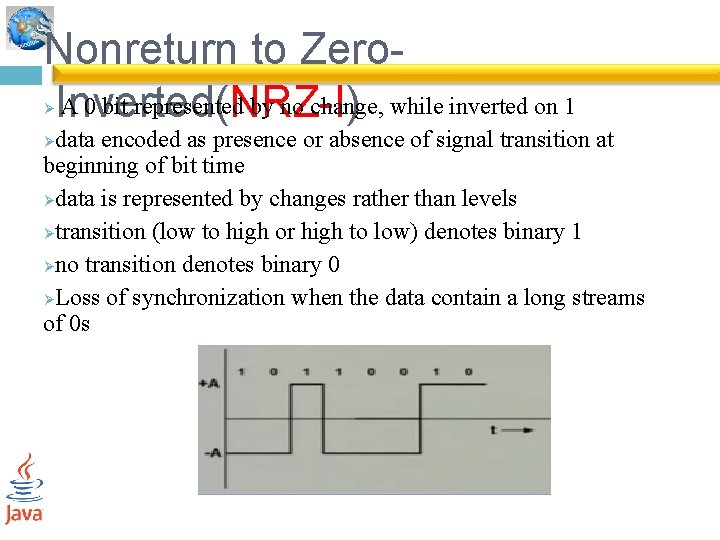Nonreturn to Zero. A 0 bit represented by no change, Inverted( NRZ-I ) while inverted on 1 Ø data encoded as presence or absence of signal transition at beginning of bit time Ødata is represented by changes rather than levels Øtransition (low to high or high to low) denotes binary 1 Øno transition denotes binary 0 ØLoss of synchronization when the data contain a long streams of 0 s Ø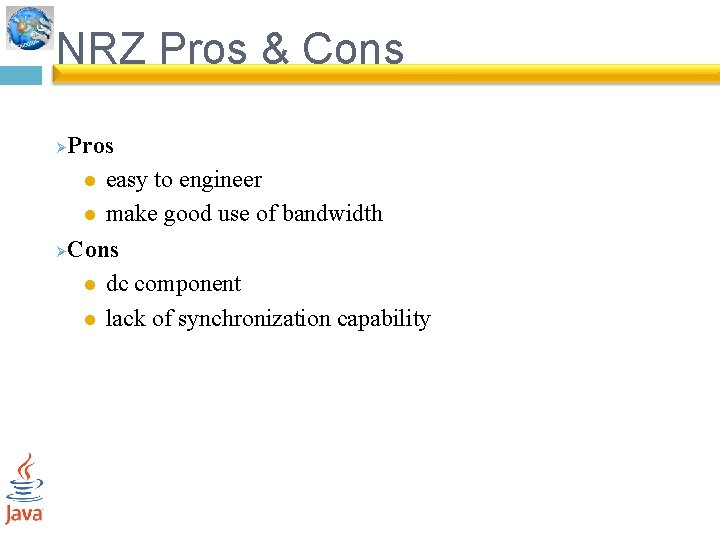NRZ Pros & Cons Pros l easy to engineer l make good use of bandwidth ØCons l dc component l lack of synchronization capability Ø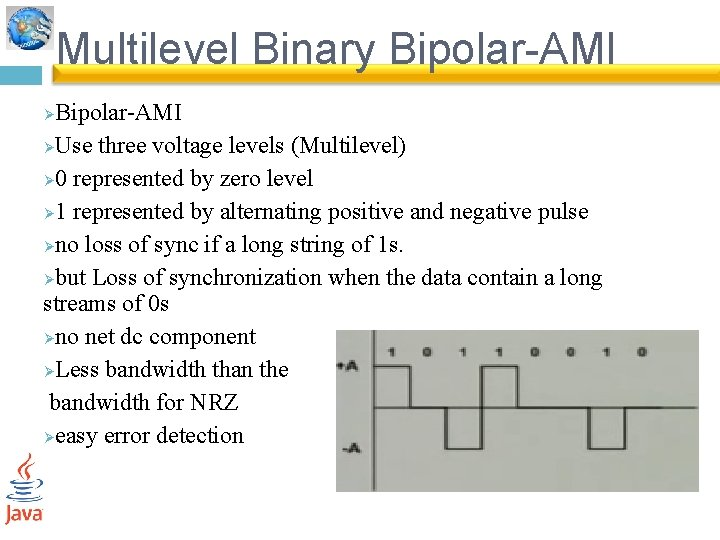Multilevel Binary Bipolar-AMI ØUse three voltage levels (Multilevel) Ø 0 represented by zero level Ø 1 represented by alternating positive and negative pulse Øno loss of sync if a long string of 1 s. Øbut Loss of synchronization when the data contain a long streams of 0 s Øno net dc component ØLess bandwidth than the bandwidth for NRZ Øeasy error detection ØMultilevel Binary Pseudoternary Bipolar ØUse three voltage levels (Multilevel) Ø 1 represented by zero level Ø 0 represented by alternating positive and negative pulse Øno loss of sync if a long string of 0 s. Øbut Loss of synchronization when the data contain a long streams of 1 s Øno advantage or disadvantage over bipolar-AMI ØMultilevel Binary Issues synchronization with long runs of 0’s or 1’s Ønot as efficient as NRZ l each signal element only represents one bit receiver distinguishes between three levels: +A, -A, 0 Ø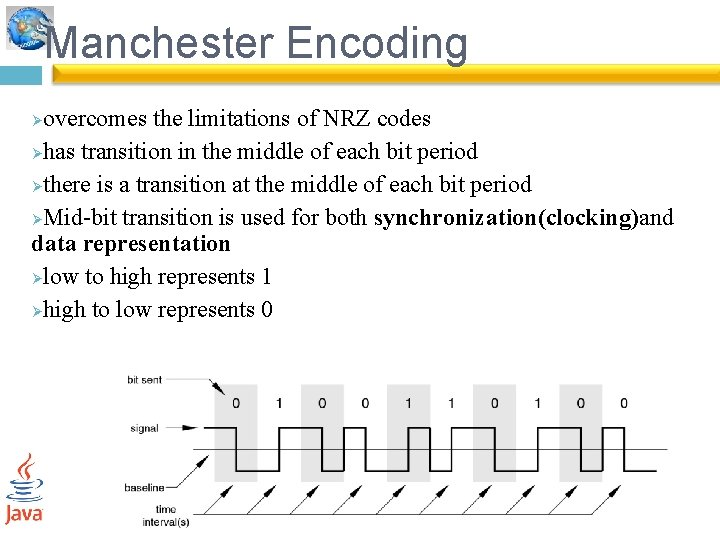Manchester Encoding overcomes the limitations of NRZ codes Øhas transition in the middle of each bit period Øthere is a transition at the middle of each bit period ØMid-bit transition is used for both synchronization(clocking)and data representation Ølow to high represents 1 Øhigh to low represents 0 Ø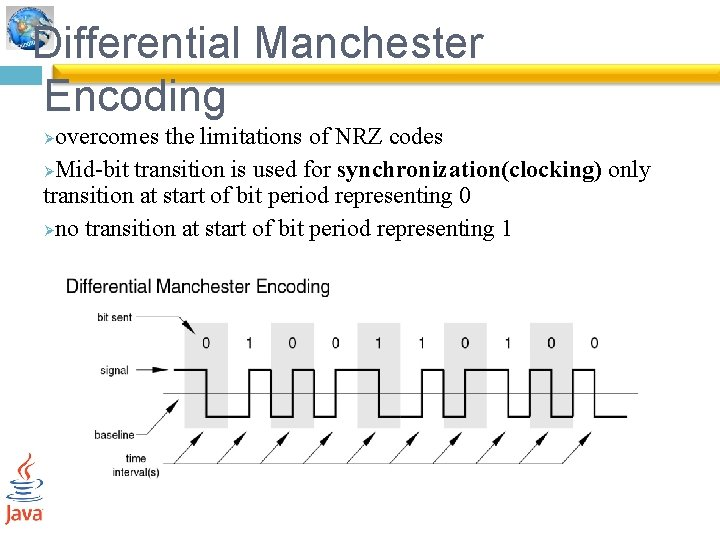Differential Manchester Encoding overcomes the limitations of NRZ codes ØMid-bit transition is used for synchronization(clocking) only transition at start of bit period representing 0 Øno transition at start of bit period representing 1 Ø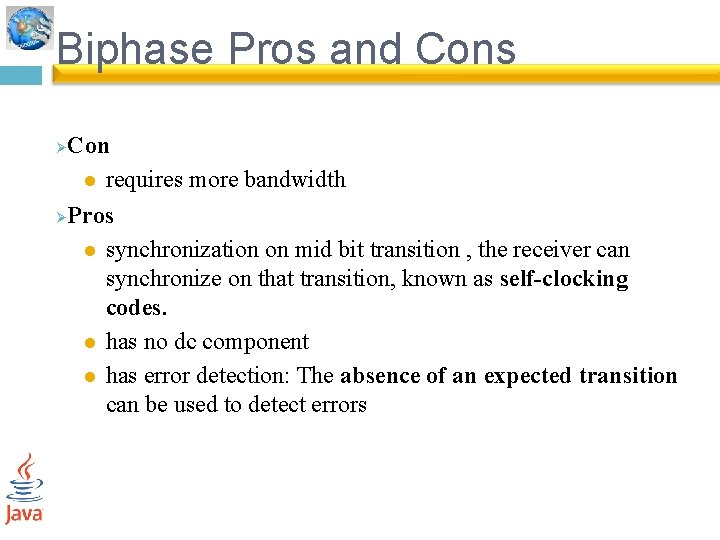Biphase Pros and Cons Con l requires more bandwidth ØPros l synchronization on mid bit transition , the receiver can synchronize on that transition, known as self-clocking codes. l has no dc component l has error detection: The absence of an expected transition can be used to detect errors Ø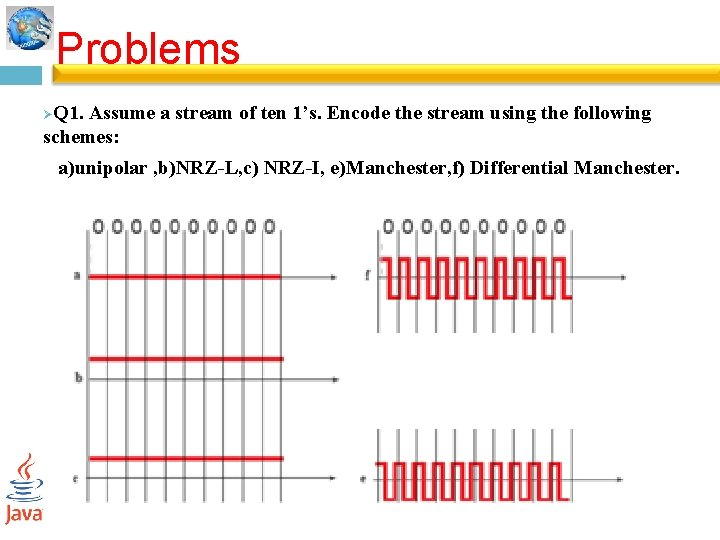Problems Q 1. Assume a stream of ten 1’s. Encode the stream using the following schemes: Ø a)unipolar , b)NRZ-L, c) NRZ-I, e)Manchester, f) Differential Manchester.Problems ØQ 2. For the Manchester encoded binary stream of the following page, extract the clock information and the data sequence. 1 0 0 1 1 1 0 0 1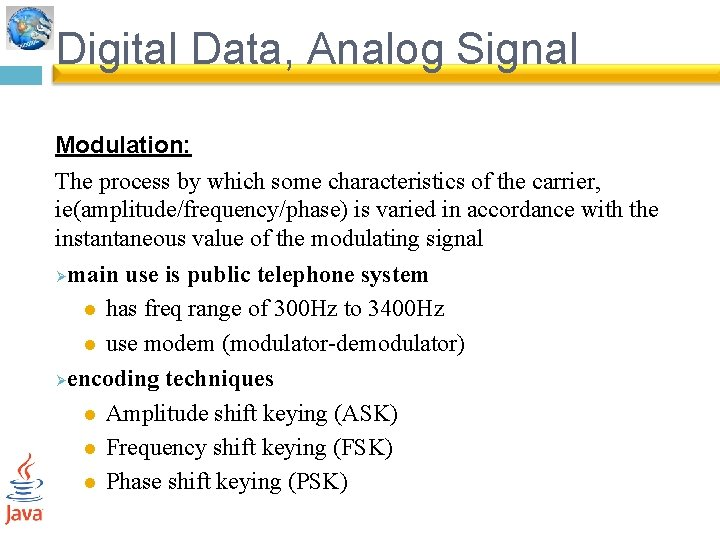Digital Data, Analog Signal Modulation: The process by which some characteristics of the carrier, ie(amplitude/frequency/phase) is varied in accordance with the instantaneous value of the modulating signal Ømain use is public telephone system l has freq range of 300 Hz to 3400 Hz l use modem (modulator-demodulator) Øencoding techniques l Amplitude shift keying (ASK) l Frequency shift keying (FSK) l Phase shift keying (PSK)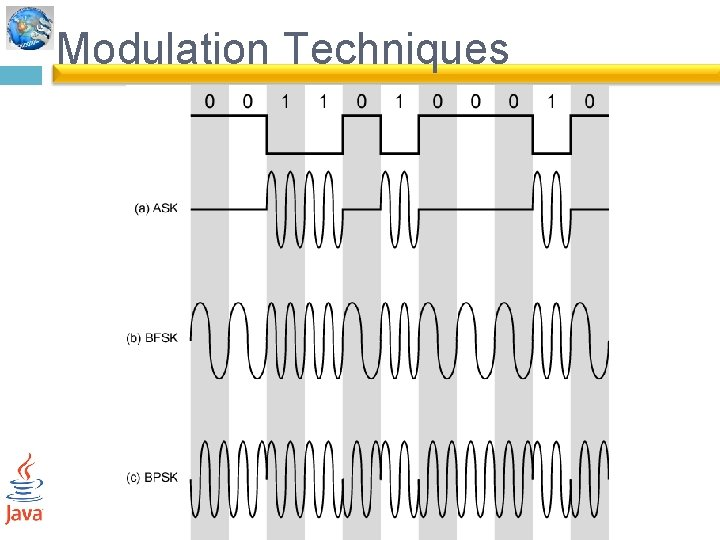Modulation TechniquesAmplitude Shift Keying Ø Values represented by two different amplitudes of the carrier. l usually have one amplitude zero susceptible to sudden gain changes Øinefficient Øused for l up to 1200 bps l very high speeds over optical fiber ØBinary Frequency Shift Keying most common is binary FSK (BFSK) Øtwo binary values represented by two different frequencies Ø less susceptible to error than ASK Øused for l up to 1200 bps l high frequency radio transmission l higher frequency on LANs using coaxial cable ØPhase Shift Keying phase of carrier signal is shifted to represent data Ø binary PSK Ø l two phases represent two binary digits differential PSK Ø l phase shifted relative to previous transmission rather than some constant reference signal l a binary 0 is represented by sending a signal burst of the same phase as the previous signal burst sent. l A binary 1 is represented by sending a signal burst of opposite phase to the preceding one.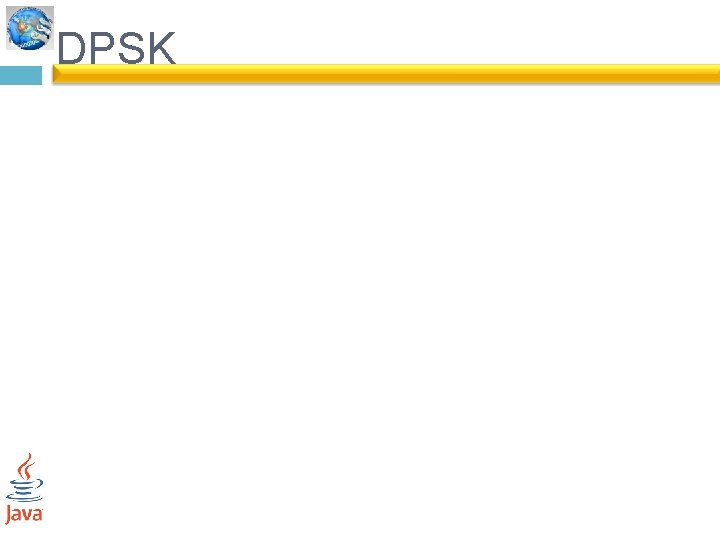DPSKQuadrature PSK get more efficient use if each signal element represents more than one bit l e. g. shifts of /2 or (90) l each element represents two bits Ø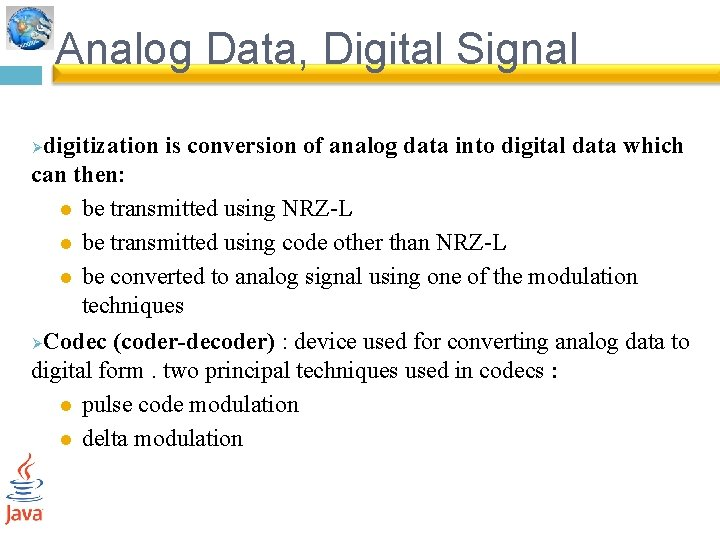Analog Data, Digital Signal digitization is conversion of analog data into digital data which can then: l be transmitted using NRZ-L l be transmitted using code other than NRZ-L l be converted to analog signal using one of the modulation techniques ØCodec (coder-decoder) : device used for converting analog data to digital form. two principal techniques used in codecs : l pulse code modulation l delta modulation ØDigitizing Analog Data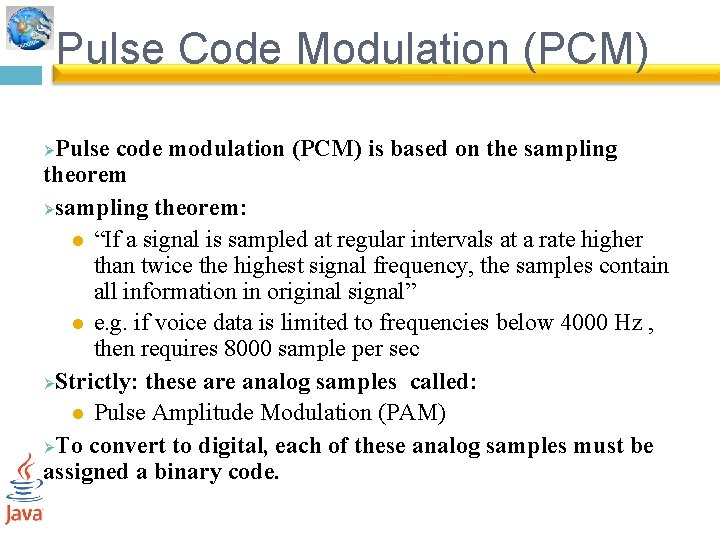Pulse Code Modulation (PCM) Pulse code modulation (PCM) is based on the sampling theorem Øsampling theorem: l “If a signal is sampled at regular intervals at a rate higher than twice the highest signal frequency, the samples contain all information in original signal” l e. g. if voice data is limited to frequencies below 4000 Hz , then requires 8000 sample per sec ØStrictly: these are analog samples called: l Pulse Amplitude Modulation (PAM) ØTo convert to digital, each of these analog samples must be assigned a binary code. ØPCM Example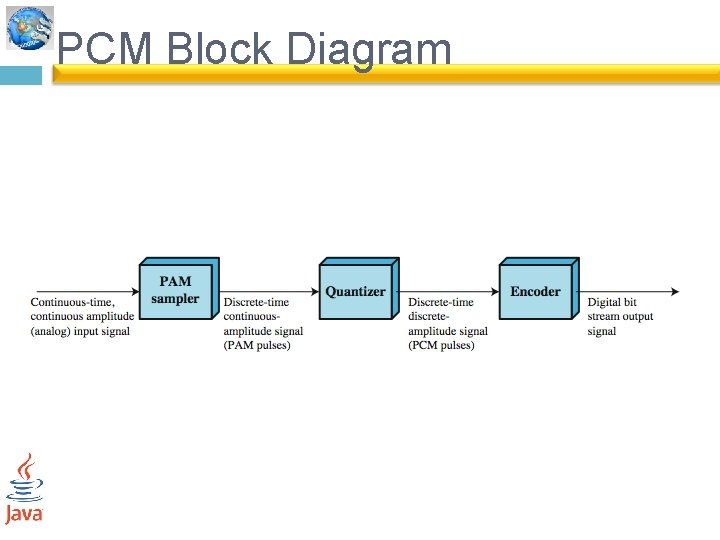PCM Block Diagram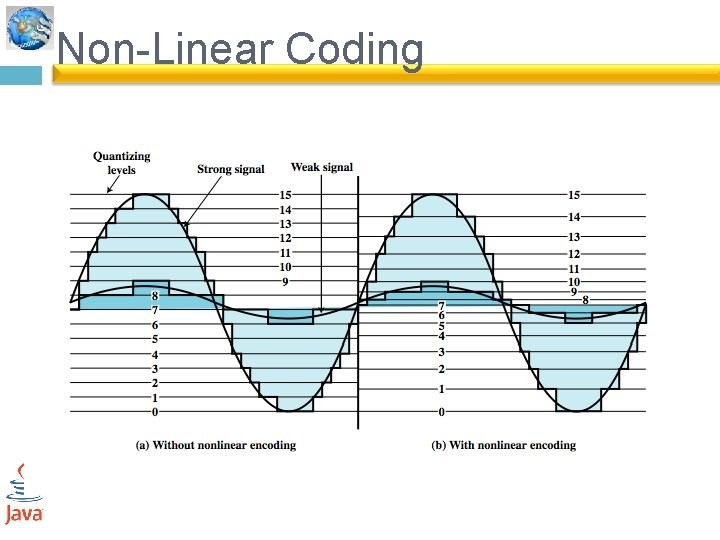Non-Linear CodingDelta Modulation analog input is approximated by a staircase function l can move up or down one level ( ) at each sample interval Øhas binary behavior l since function only moves up or down at each sample interval l hence can encode each sample as single bit l 1 for up or 0 for down Ø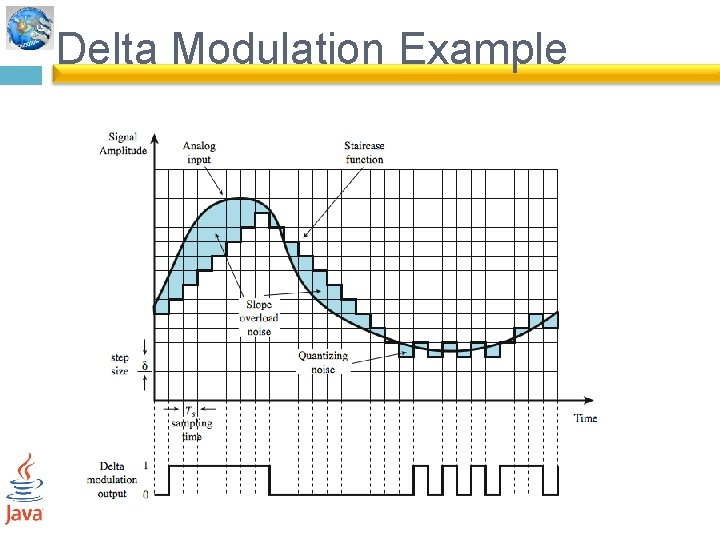Delta Modulation ExamplePCM verses Delta Modulation DM has simplicity compared to PCM Øbut has worse SNR ØPCM preferred to DM for analog signals Ø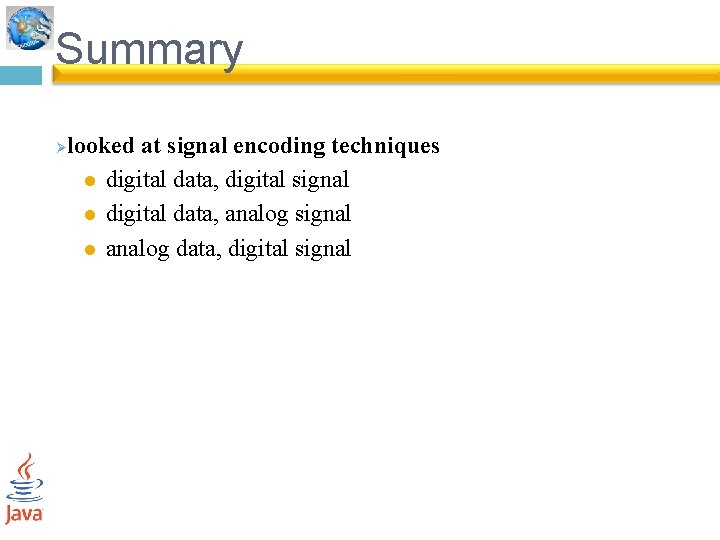Summary Ø looked at signal encoding techniques l digital data, digital signal l digital data, analog signal l analog data, digital signal